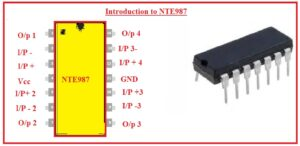Hello, guys welcome to the new post. we will discuss Introduction to NTE987. It is less power-consuming quad operational amplifier’s ability to operate at three volts. It comes with a differential input stage and can work at different values of volts ranging from three volts to thirty two volts.

In this post, we will cover the basics of this module with details, a working pinout, and some other points. So let’s get started Introduction to NTE987.

### Introduction to NTE987

• The NTE987 is quad operation amplifier having differential inputs. It has certain benefits over the normal amplifiers in single supply applications.
• It has the ability to operate on the volts level from three to thirty volts.
• It has a negative supply for common-mode input that reduces the use of different components for exterior-based circuits.
• The ranges for output volts comprise the negative power supply.
• It comes with a voltage gain of one hundred and CMRR ratio is about seventy.
• Its O/P pins of operational amplifiers come with a shirt circuit structure. The least input-biased current for this module is 0.25 microamperes.### NTE987 Pinout

• The main pinout of NTE987 are described here
• Pin 1 is the output of first operational amplifier
• Pin 2 is negative input of inverting operational amplifier
• Pin 3 is the positive input of non-inverting input of first amplifier
• Pin 4 is Vcc positive supply given here
• Pin 5 is input positive of non-inventing input operational amplifier
• Pin 6 is the negative input of operational amplifier 2
• Pin 7 is output of the second amplifier
• Pin 8 is output of the third operational amplifier
• Pin 9 is negative terminal of third operational amplifier
• Pin 10 is positive input of non-iverting of third operational amplifier
• Pin 11 is Ground
• Pin 12 is input positive of fourth operational amplifier
• Pin 13 Inverting  input of 4th amplifies
• Pin 14 is the output of 4th amplifies

### NTE987 Features

• It comes 4 packaging that amplifies
• Its packaging is dual in line fourteen pin
• Its input biasing current is 0.25 microamperes
• Its CMRR value is seventy
• The gain value of one hundred decibels
• Its output volts sometimes negative and work at the negative supply
• Its supply current value is three milliamperes
• Volts range is three to thirty-two volts

### NTE987 Applications• Its common applications are explained here
• It used as a comparator
• Used in dc gain circuits
• Since consists of operational amplifiers so used as differentiators, summer, adders.
• Used in filter circuits and transducers

That is all about the NTE987 all paramerts has been explained. If you have any further quey ask in comments. Thanks for reading have a nice day